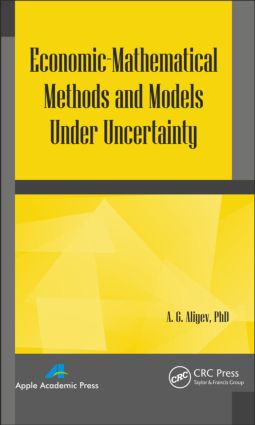Economic-Mathematical Methods and Models under Uncertainty

1st Edition

302 pages | 32 B/W Illus.

Purchasing Options:\$ = USD
Hardback: 9781926895567
pub: 2013-12-07
SAVE ~\$33.00
\$165.00
\$132.00
x
eBook (VitalSource) : 9780429172809
pub: 2013-12-07
from \$28.98

FREE Standard Shipping!

Description

In this book on mathematical programming, the postulate spacial-time certainty of economic process at uncertainty conditions in finite-dimensional vector space and the principle piecewise-linear homogeneity of economic process at uncertainty conditions in finite-dimensional vector space are first suggested. A special theory on constructing piecewise-linear economic-mathematical models was developed, and a criterion of multivariate prediction of economic process and their control at uncertainty conditions in a finite-dimensional vector space was suggested.

A packet of numerical programs for computer simulation in constructing and multivariate prediction of economic state with the help of n-element piecewise-linear economic-mathematical models with regard to the uncertainty factors effect in m-dimensional vector space is also suggested.

This book is intended for students of economic and administrative specialties as well as for research associates in the sphere of economic-mathematical methods, management, and banking.

Brief Information on Finite-Dimensional Vector Space and its Application in Economics

Bases of Piecewise-Linear Economic-Mathematical Models with Regard to Influence of Unaccounted Factors in Finite-Dimensional Vector Space

Piecewise Linear Economic-Mathematical Models with Regard to Unaccounted Factors Influence in Three-Dimensional Vector Space

Piecewise-Linear Economic-Mathematical Models with Regard to Unaccounted Factors Influence on a Plane

Bases of Software for Computer Simulation and Multivariant Prediction of Economic Even at Uncertainty Conditions on the Base of N-Component Piecewise-Linear Economic-Mathematical Models in M-Dimensional Vector Space

Index